Criteria of congruency of triangles

Chapter 7 Class 7 Congruence of Triangles
Concept wise

Suppose, we have two triangles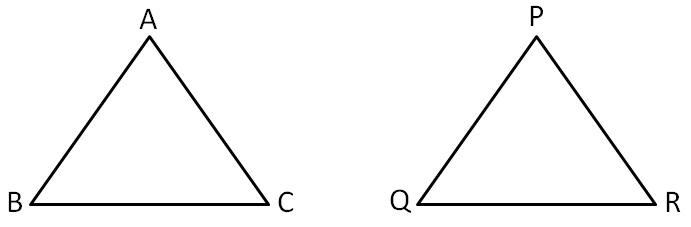To prove ∆ABC ≅ ∆PQR,

We use different congruency criteria

They are

• SSS – Side Side Side
• SAS − Side Angle Side
• ASA – Angle Side Angle
• AAS − Same as ASA
• AAA – Angle Angle Angle (Check Why AAA is not a congruence )
• RHS − Right-angle Hypotenuse side

Let’s discuss them

#### SSSIn ∆ABC & ∆PQR

AB = PQ                (Both are 5)

BC = QR               (Both are 4)

AC = PR               (Both are 3)

∴ ∆ABC ≅ ∆PQR   (SSS Congruence Rule)

For more details,

Please check SSS Explanation  & proof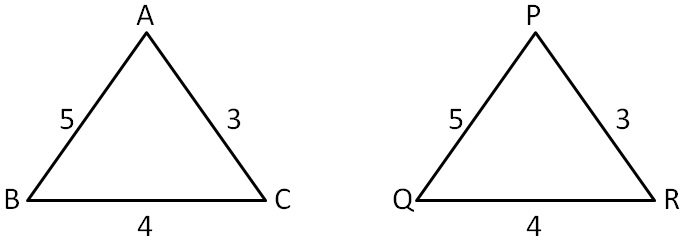Note :

Here ∆ABC ∆PQR

not ∆ABC ∆QRP

Order of writing the triangle is important

#### SAS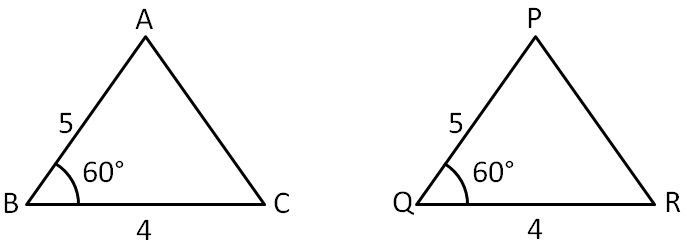In ∆ABC & ∆PQR

AB = PQ                  ( Both are 5 )

∠B  = ∠Q                 ( Both are 60° )

BC = QR                 ( Both are 4 )

∴ ∆ABC ≅ ∆PQR    ( SAS Congruence Rule )

For more details,

Please check SAS Explanation  & proof

#### ASA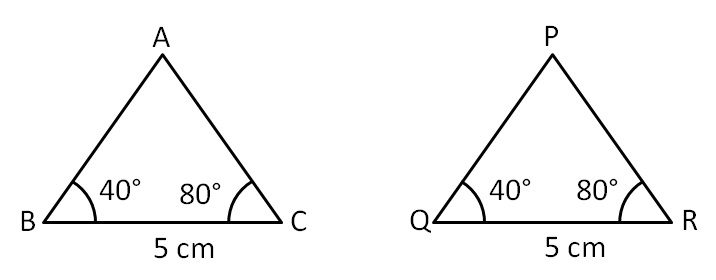In ∆ABC & ∆PQR

∠B = ∠Q         ( Both are 40 °)

BC  = QR       ( Both are 5 cm )

∠C = ∠R        ( Both are 80 °)

∴ ∆ABC ≅ ∆PQR

For more details,

Please check ASA Explanation & proof

### RHS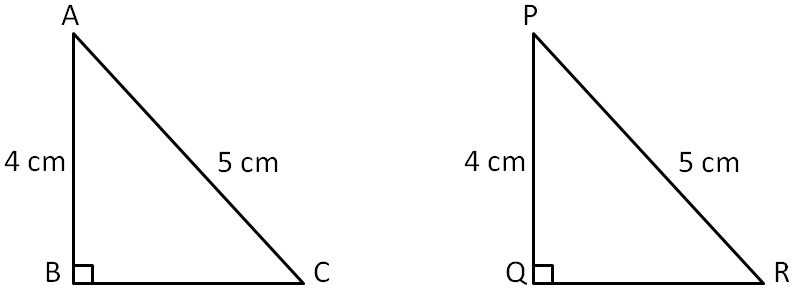In ∆ABC & ∆PQR

∠B = ∠Q      ( Right angle, both 90 °)

AC  = PR    ( Hypotenuse, both 5 cm )

AB = PQ     (Side , both 4 cm )

∴ ∆ABC ≅ ∆PQR        ( RHS Congruency rule )

For more details,

Please check RHS Explanation  & proof

Get live Maths 1-on-1 Classs - Class 6 to 12